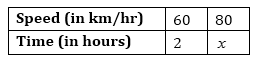# A car takes 2 hours to reach a destination by travelling at the speed of 60 km/hr. How long will it take when the car travels at the speed of 80 km/hr?

Solution

Let the number of machines required be x.The number of machines and the number of days are inversely proportional.

Therefore, from inverse proportionality property:-

60/80 = x/2

60×2 = 80x

x = 60×2/80

x = 120 / 80

x = (3/2) hours.

Therefore, time taken to reach destination, when car is traveling at Speed of 80 Km/h is (3/2) hours.(2)(1)